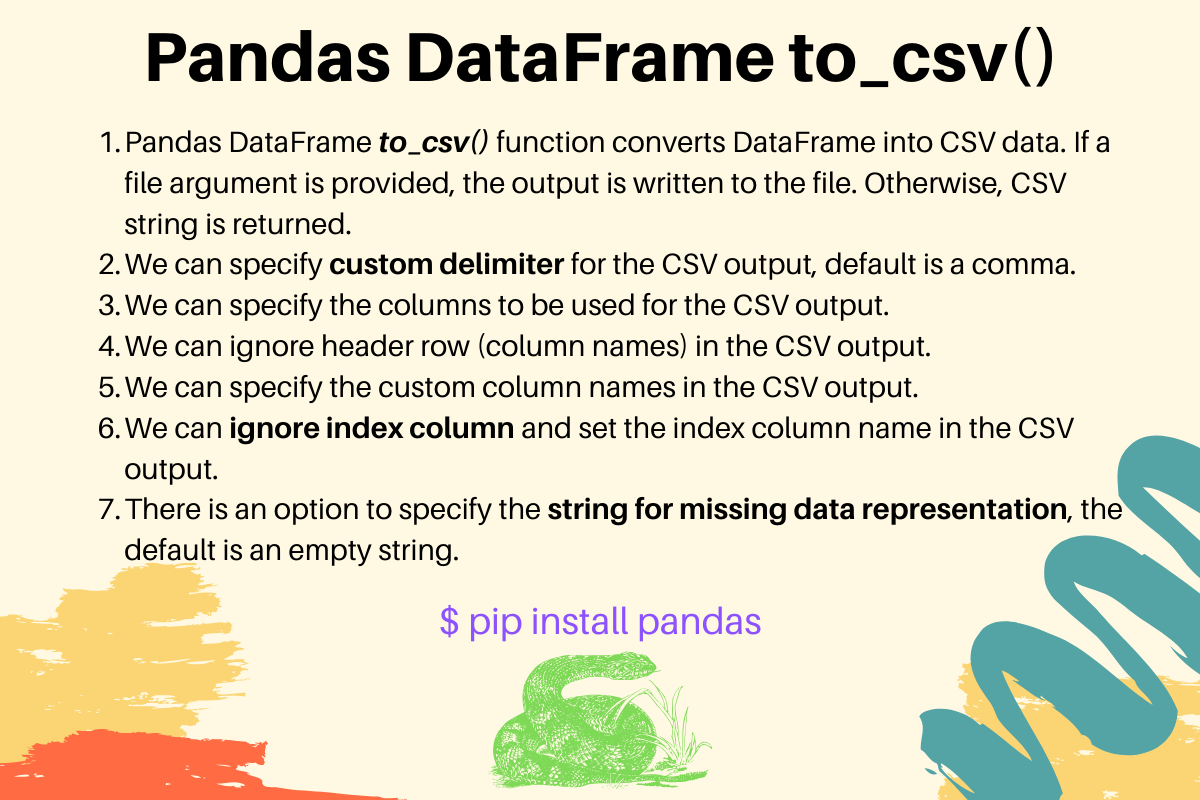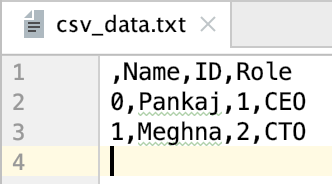# Pandas to_csv() - Convert DataFrame to CSV

Published on August 3, 2022By Pankaj
Developer and author at DigitalOcean.While we believe that this content benefits our community, we have not yet thoroughly reviewed it. If you have any suggestions for improvements, please let us know by clicking the “report an issue“ button at the bottom of the tutorial.

Pandas DataFrame to_csv() function converts DataFrame into CSV data. We can pass a file object to write the CSV data into a file. Otherwise, the CSV data is returned in the string format.

## Pandas DataFrame to_csv() Syntax

The syntax of DataFrame to_csv() function is:

``````def to_csv(
self,
path_or_buf=None,
sep=",",
na_rep="",
float_format=None,
columns=None,
index=True,
index_label=None,
mode="w",
encoding=None,
compression="infer",
quoting=None,
quotechar='"',
line_terminator=None,
chunksize=None,
date_format=None,
doublequote=True,
escapechar=None,
decimal=".",
)
``````

Some of the important parameters are:

• path_or_buf: the file object to write the CSV data. If this argument is not provided, the CSV data is returned as a string.
• sep: the delimiter for the CSV data. It should be a string of length 1, the default is a comma.
• na_rep: string representing null or missing values, default is empty string.
• columns: a sequence to specify the columns to include in the CSV output.
• header: the allowed values are boolean or a list of string, default is True. If False, the column names are not written in the output. If a list of string, it’s used to write the column names. The length of the list of string should be the same as the number of columns being written in the CSV file.
• index: if True, index is included in the CSV data. If False, the index value is not written in the CSV output.
• index_label: used to specify the column name for index.

## Pandas DataFrame to CSV Examples

Let’s look at some common examples of using to_csv() function to convert DataFrame to CSV data.

### 1. Converting DataFrame to CSV String

``````import pandas as pd

d1 = {'Name': ['Pankaj', 'Meghna'], 'ID': [1, 2], 'Role': ['CEO', 'CTO']}

df = pd.DataFrame(d1)

print('DataFrame:\n', df)

# default CSV
csv_data = df.to_csv()
print('\nCSV String:\n', csv_data)
``````

Output:

``````DataFrame:
Name  ID Role
0  Pankaj   1  CEO
1  Meghna   2  CTO

CSV String:
,Name,ID,Role
0,Pankaj,1,CEO
1,Meghna,2,CTO
``````

### 2. Specifying Delimiter for the CSV Output

``````csv_data = df.to_csv(sep='|')
print(csv_data)
``````

Output:

``````|Name|ID|Role
0|Pankaj|1|CEO
1|Meghna|2|CTO
``````

If the specified delimiter length is not 1, TypeError: “delimiter” must be a 1-character string is raised.

### 3. Selecting only few columns for CSV Output

``````csv_data = df.to_csv(columns=['Name', 'ID'])
print(csv_data)
``````

Output:

``````,Name,ID
0,Pankaj,1
1,Meghna,2
``````

Notice that the index is not considered to be a valid column.

### 4. Ignoring Header Row in the CSV Output

``````csv_data = df.to_csv(header=False)
print(csv_data)
``````

Output:

``````0,Pankaj,1,CEO
1,Meghna,2,CTO
``````

### 5. Setting Custom Column Names in the CSV

``````csv_data = df.to_csv(header=['NAME', 'ID', 'ROLE'])
print(csv_data)
``````

Output:

``````,NAME,ID,ROLE
0,Pankaj,1,CEO
1,Meghna,2,CTO
``````

Again the index is not considered as the column of DataFrame object.

### 6. Skipping Index Column in CSV Output

``````csv_data = df.to_csv(index=False)
print(csv_data)
``````

Output:

``````Name,ID,Role
Pankaj,1,CEO
Meghna,2,CTO
``````

### 7. Setting Index Column Name in the CSV

``````csv_data = df.to_csv(index_label='Sl No.')
print(csv_data)
``````

Output:

``````Sl No.,Name,ID,Role
0,Pankaj,1,CEO
1,Meghna,2,CTO
``````

### 8. Converting DataFrame to CSV File

``````with open('csv_data.txt', 'w') as csv_file:
df.to_csv(path_or_buf=csv_file)
``````

We are using with statement to open the file, it takes care of closing the file when the with statement block execution is finished. This code snippet will create a CSV file with the following data.### 9. Null, NA, or Missing Data Representation in the CSV Output

``````import pandas as pd

d1 = {'Name': ['Pankaj', 'Meghna'], 'ID': [1, pd.NaT], 'Role': [pd.NaT, 'CTO']}
df = pd.DataFrame(d1)
print('DataFrame:\n', df)

csv_data = df.to_csv()
print('\nCSV String:\n', csv_data)

csv_data = df.to_csv(na_rep="None")
print('CSV String with Null Data Representation:\n', csv_data)
``````

Output:

``````DataFrame:
Name   ID Role
0  Pankaj    1  NaT
1  Meghna  NaT  CTO

CSV String:
,Name,ID,Role
0,Pankaj,1,
1,Meghna,,CTO

CSV String with Null Data Representation:
,Name,ID,Role
0,Pankaj,1,None
1,Meghna,None,CTO
``````